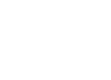# Quants Questions : LCM & HCF Set 2

Hello Aspirants. Welcome to Online Quantitative Aptitude Section with explanation in AffairsCloud.com. Here we are creating question sample in LCM & HCF, which is common for all the IBPS,SBI exam and other competitive exams. We have included Some questions that are repeatedly asked in bank exams !!!

1. If the product of  two numbers is 2496 and HCF is 8,then the ratio of HCF and LCM is
A)1:32
B)39:1
C)1:39
D)4:63
E)None of these
Explanation:
HCF = 8
LCM = Product/HCF
Product of the 2 number = 2496
HCF:LCM = 8 : (2496/8) => 8 : 312 => 1: 39

2. The greatest possible length  which can be used to measure exactly the lengths 1m 92cm,3m 84cm ,23m 4cm
A)23
B)32
C)36
D)34
E)None of these
Explanation:
192 = 4^2×2^2×3
384 = 4^2×2^2×6
2304 = 4^2×2×6^2
HCF = 4^2×2 = 16×2 = 32

3. HCF of 4/3, 8/6, 36/63 and 20/42
A)4/126
B)4/8
C)4/36
D)4/42
E)None of these
Explanation:
HCF of numerator(4,8,36,20) = 4
LCM of denominator(3,6,63,42) = 126

4. Find the LCM of 3/8, 9/32, 33/48, 18/72
A)3/8
B)8/33
C)198/8
D)8/3
E)None of these
Explanation :
LCM of numerator(3,9,33,18) = 198
HCF of denominator(8,32,48,72) = 8

5. The HCF of 2511 and 3402 is
A)31
B)42
C)76
D)81
E)None of these
Explanation :
2511 = 81×31
3402 = 81×42
Hence HCF is 81

6. A gardener had a number of shrubs to plant in rows. At first he tried to plant 8,then 12 and then 16 in a row but he had always 3 shrubs left with him. On trying 7 he had none left. Find the total number of shrubs.
A)154
B)147
C)137
D)150
E)None of these
Explanation :7. If m and n are two whole numbers and if m^n= 49. Find n^m, given that n ≠ 1
A)118
B)94
C)561
D)128
E)None of these
Explanation :
49 = 7^2 = m^n
n^m = 2^7 = 128

8. What will be the least number which when doubled will be exactly divisible by 12,18,21 and 30?
A)630
B)360
C)603
D)306
E)None of these
Explanation :
LCM of 12,18,21 and 30 = 1260
Doubled (divide by 2) = 1260/2 = 630

9. HCF and LCM of two numbers are 11 and 385 .If one number lies between 75 and 125 then that number is
A)123
B)73
C)77
D)154
E)None of these
Explanation :
Product of the two number = 11a×11b = 4235
11a×11ab = 4235/121 = 35
35 = 7 × 5 (co prime)
77 × 11 = 77

10. If the L.C.M of x and y is z, their H.C.F is
A)xy/z
B)xyz
C)(x + y)/z
D)z/xy
E)None of these